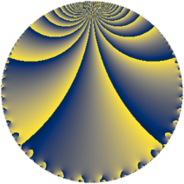# Properties

 Label 784.4.fLevel $784$ Weight $4$ Character orbit 784.f Rep. character $\chi_{784}(783,\cdot)$ Character field $\Q$ Dimension $60$ Newform subspaces $10$ Sturm bound $448$ Trace bound $9$

# Related objects

## Defining parameters

 Level: $$N$$ $$=$$ $$784 = 2^{4} \cdot 7^{2}$$ Weight: $$k$$ $$=$$ $$4$$ Character orbit: $$[\chi]$$ $$=$$ 784.f (of order $$2$$ and degree $$1$$) Character conductor: $$\operatorname{cond}(\chi)$$ $$=$$ $$28$$ Character field: $$\Q$$ Newform subspaces: $$10$$ Sturm bound: $$448$$ Trace bound: $$9$$ Distinguishing $$T_p$$: $$3$$

## Dimensions

The following table gives the dimensions of various subspaces of $$M_{4}(784, [\chi])$$.

Total New Old
Modular forms 360 60 300
Cusp forms 312 60 252
Eisenstein series 48 0 48

## Trace form

 $$60q + 540q^{9} + O(q^{10})$$ $$60q + 540q^{9} - 2004q^{25} - 168q^{29} + 504q^{37} + 1176q^{53} + 2376q^{57} + 552q^{65} + 3924q^{81} - 2712q^{85} - 4248q^{93} + O(q^{100})$$

## Decomposition of $$S_{4}^{\mathrm{new}}(784, [\chi])$$ into newform subspaces

Label Dim. $$A$$ Field CM Traces $q$-expansion
$$a_2$$ $$a_3$$ $$a_5$$ $$a_7$$
784.4.f.a $$2$$ $$46.257$$ $$\Q(\sqrt{-3})$$ None $$0$$ $$-14$$ $$0$$ $$0$$ $$q-7q^{3}+9\zeta_{6}q^{5}+22q^{9}-7\zeta_{6}q^{11}+\cdots$$
784.4.f.b $$2$$ $$46.257$$ $$\Q(\sqrt{-3})$$ None $$0$$ $$-2$$ $$0$$ $$0$$ $$q-q^{3}+3\zeta_{6}q^{5}-26q^{9}-15\zeta_{6}q^{11}+\cdots$$
784.4.f.c $$2$$ $$46.257$$ $$\Q(\sqrt{-3})$$ None $$0$$ $$2$$ $$0$$ $$0$$ $$q+q^{3}+3\zeta_{6}q^{5}-26q^{9}+15\zeta_{6}q^{11}+\cdots$$
784.4.f.d $$2$$ $$46.257$$ $$\Q(\sqrt{-3})$$ None $$0$$ $$14$$ $$0$$ $$0$$ $$q+7q^{3}-9\zeta_{6}q^{5}+22q^{9}-7\zeta_{6}q^{11}+\cdots$$
784.4.f.e $$4$$ $$46.257$$ 4.0.2048.2 $$\Q(\sqrt{-1})$$ $$0$$ $$0$$ $$0$$ $$0$$ $$q+(-\beta _{1}-5\beta _{2})q^{5}-3^{3}q^{9}+(4\beta _{1}+21\beta _{2}+\cdots)q^{13}+\cdots$$
784.4.f.f $$4$$ $$46.257$$ $$\Q(\sqrt{-3}, \sqrt{7})$$ None $$0$$ $$0$$ $$0$$ $$0$$ $$q+\beta _{1}q^{3}-5\beta _{2}q^{5}-20q^{9}-15\beta _{3}q^{11}+\cdots$$
784.4.f.g $$6$$ $$46.257$$ 6.0.$$\cdots$$.1 None $$0$$ $$-14$$ $$0$$ $$0$$ $$q+(-2+\beta _{2})q^{3}-\beta _{3}q^{5}+(26-\beta _{1}+\cdots)q^{9}+\cdots$$
784.4.f.h $$6$$ $$46.257$$ 6.0.$$\cdots$$.1 None $$0$$ $$14$$ $$0$$ $$0$$ $$q+(2-\beta _{2})q^{3}+\beta _{3}q^{5}+(26-\beta _{1}-\beta _{2}+\cdots)q^{9}+\cdots$$
784.4.f.i $$8$$ $$46.257$$ 8.0.$$\cdots$$.32 None $$0$$ $$0$$ $$0$$ $$0$$ $$q+\beta _{3}q^{3}+(2\beta _{2}+\beta _{4})q^{5}+(43+3\beta _{5}+\cdots)q^{9}+\cdots$$
784.4.f.j $$24$$ $$46.257$$ None $$0$$ $$0$$ $$0$$ $$0$$

## Decomposition of $$S_{4}^{\mathrm{old}}(784, [\chi])$$ into lower level spaces

$$S_{4}^{\mathrm{old}}(784, [\chi]) \cong$$ $$S_{4}^{\mathrm{new}}(28, [\chi])$$$$^{\oplus 6}$$$$\oplus$$$$S_{4}^{\mathrm{new}}(112, [\chi])$$$$^{\oplus 2}$$$$\oplus$$$$S_{4}^{\mathrm{new}}(196, [\chi])$$$$^{\oplus 3}$$